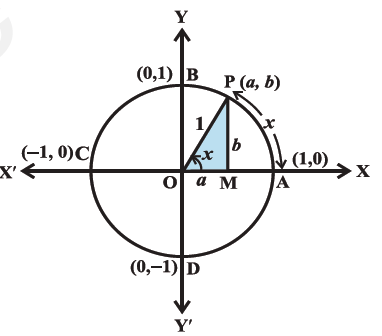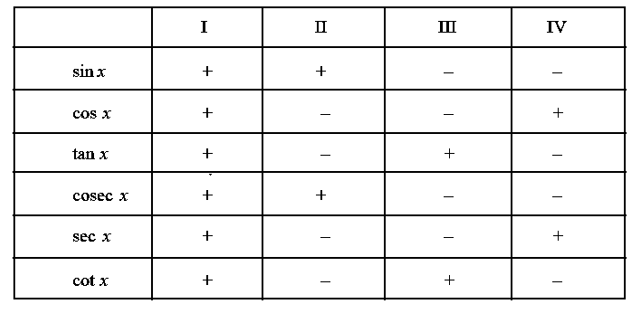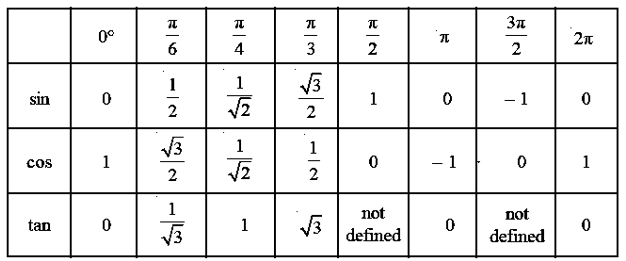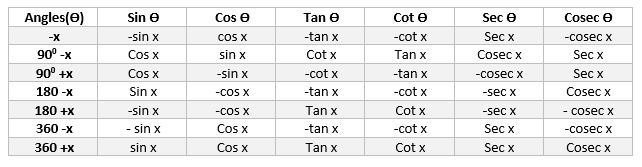# Trigonometric functions

## Trigonometric functions:

Consider a unit circle with centre at the origin O and Let P be any point on the circle with P(a,b). And let call the angle x We use the coordinates of P to define the cosine of the angle and the sine of the angle. Specifically, the x-coordinate of B is the cosine of the angle, and the y-coordinate of B is the sine of the angle. Also it is clear
a2+b2=1
cos2x +sin2x =1### Properties of Trigonometric functions

1. Sine and cosine are periodic functions of period $360^{\circ}$, that is, of period $2\pi$. That's because sines and cosines are defined in terms of angles, and you can add multiples of $360^{\circ}$, or $2\pi$, and it doesn't change the angle. Thus, for any angle x
$sin(x+2 \pi)= sin (x)$ and $cos(x+2 \pi)= cos(x)$
or we can say that
$sin (2n \pi + x) = sin x$, $n\in Z$ , $cos (2n \pi + x) = cos x$, $n\in Z$
Where Z is the set of all integers
2. sin x = 0 implies $x = n \pi$ , where n is any integer
cos x = 0 implies $x = (2n + 1)\frac {\pi}{2}$
3. The other trigonometric function are defined as
$cosec(x)= \frac {1}{sin (x)}$ where $x \neq n \pi$, where n is any integer
$sec(x)=\frac {1}{cos (x)}$ where $x \neq (2n + 1)\frac {\pi}{2}$ where n is any integer
$tan(x)=\frac {sin(x)}{cos(x)}$ where $x \neq (2n + 1)\frac {\pi}{2}$ where n is any integer
$cot(x)=\frac {cos(x)}{sin(x)}$ where $x \neq n \pi$, where n is any integer
4. Trigonometric Functions identities
For all real x
$sin ^2(x)+cos ^2(x)=1$
$1+ tan ^2(x)=sec ^2(x)$
$1+ cot ^2(x)=cosec ^2(x)$
5. What is is Odd function and Even Function
We have come across these adjectives 'odd' and 'even' when applied to functions, but it's important to know them. A function f is said to be an odd function
if for any number x, f(-x) = -f(x).
A function f is said to be an even function if for any number x, f(-x) = f(x).
Many functions are neither odd nor even functions, but some of the most important functions are one or the other.
Example:
Any polynomial with only odd degree terms is an odd function, for example, f(x) = 2x7 + 9x5 - x. (Note that all the powers of x are odd numbers.)
Similarly, any polynomial with only even degree terms is an even function. For example, f(x) = 6x8 - 6x2 - 5.
Based on above definition we can call Sine is an odd function, and cosine is even
sin (-x) = -sin x, and
cos (-x) = cos x.
These facts follow from the symmetry of the unit circle across the x-axis. The angle -x is the same angle as x except it's on the other side of the x-axis. Flipping a point (x,y) to the other side of the x-axis makes it into (x,-y), so the y-coordinate is negated, that is, the sine is negated, but the x-coordinate remains the same, that is, the cosine is unchanged.
6. Sign of the Trigonometric functions Now since in unit circle
$-1 \leq a \leq 1$
$-1 \leq b \leq 1$
It follows that for all x
$-1 \leq sin(x) \leq 1$
$-1 \leq cos(x) \leq 1$
Also We know from previous classes,
(i) a,b are both positive in Ist quadrant i.e $0< x < \frac {\pi}{2}$ , It implies that sin is positive and cos is positive
(ii) a is negative and b is positive in IInd quadrant i.e $\frac {\pi}{2} < x < \pi$ ,It implies that sin is negative and cos is positive
(iii) a and b both are negative in III quadrant ie. $\pi < x < \frac {3 \pi}{2}$ ,It implies that sin is negative and cos is negative
(iv) a is positive and b is negative in IV quadrant i,.e $\frac {3 \pi}{2} < x < 2 \pi$ It implies that sin is positive and cos is negative
Similarly sign can be obtained for other functions
This can be summarized in table as belowThis can be remember as ALL SILVER TEA CUPS
ALL -> ALL are positive in Ist quadrant
SILVER -> Sin function and its reciprocal function are positive
TEA -> Tangent function and its reciprocak function are positive
CUPS -> Cos function and its reciprocal function are positive
7. The common values are same as trigonometric ratios for those angles8. The relation for complementary angles and ssupplementary angles are given below